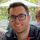cancel
Showing results for
Did you mean:## Help with a measure

Hi guys, I'm a big newbie to Power BI, and I need help with a measure:

I need to subtract 2 measures, if the result it gives me is less than or equal to zero I need it to return 0, if the date is earlier than today's date I need to return zero, and if it is not fulfilled that I return the result of difference. If someone can help me, I would appreciate it.

Thanks a lot

3 REPLIES 3Thanks for the answer but it has not worked for me gives me the following error:Thank youSuper User

You're missing a closing bracket on line 5.Super User

Hi,

`Subtract = VAR _ameasure = SELECTEDVALUE(A value)VAR _bmeasure = SELECTEDVALUE(B value)VAR _date = SELECTEDVALUE(date)VAR _result = IF(_ameausre - _bmeasure <= 0, 0, IF(_date < TODAY(), 0, _ameasure - _bmeasure))RETURN_result`